Oxidation numbers So far we have defined redox (reduction/oxidation) reactions in terms of electron transfer. It is easy to determine the oxidant and reductant in reactions such as in the one below between magnesium and oxygen.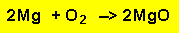It is easy to see that magnesium atoms have been oxidised (lost electrons) to form magnesium ions with a charge of 2+. While oxygen atoms have taken electrons to form oxygen ions with a charge of 2-. Here we can decide which species is the oxidant and which is the reductant according to the flow of electrons. What about reactions that do not involve ions? Reactions such as the formation of water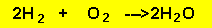In this reaction electrons are shared not transferred and there is no clear formation of charge. The reaction between hydrogen and oxygen is clearly a redox reaction. Clearly we need to broaden our definition of redox reactions. This broader definition involves the assignment of oxidation numbers to atoms taking part in the reaction. A few rules apply. Rule 1) The oxidation number of all substances in their elemental form is zero.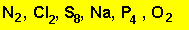The oxidation number of all the atoms above is zero. Rule 2) The oxidation number of all monoatomic(single atom) ions is the charge on the ion.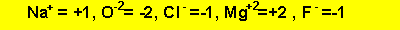Rule 3) The oxidation number of oxygen is -2. Exceptions are peroxides where it is -1 and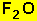where it is +2. Rule 4) Hydrogen is +1 except in metal hydrides where it is -1. Rule 5) In polyatomic ions and molecules the sum of the oxidation numbers of each atom is equal to the charge on the ion or molecule. Example 1)What is the oxidation number of manganese in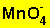Mn + 4 X -2 = -1 Mn = 8 -1 =7 2) What is the oxidation number of Cr in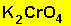(scan the formula for more information) The oxidation number of K=1 and for O = -2. The overall charge is 0. 2 X 1 + Cr + 4 X -2 = 0 Cr = 6 3) What is the oxidation number of manganese in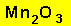(scan the formula for more information) 2X Mn + 3 X -2 =0 2Mn = 6 Mn =3 Exercises. 1) Find the oxidation number of carbon in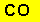and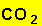2) Find the oxidation number of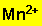3) Find the oxidation number of the sulfur in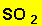and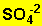4) Find the oxidation number of Cl in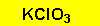and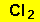Scan the formulae for the answer